# A Complete Machine Learning Project Walk-Through in PythonA Complete Machine Learning Project Walk-Through in Python: Putting the machine learning pieces together; Model Selection, Hyperparameter Tuning, and Evaluation; Interpreting a machine learning model and presenting results

## A Complete Machine Learning Project Walk-Through in Python (Part One): Putting the machine learning pieces together

Reading through a data science book or taking a course, it can feel like you have the individual pieces, but don’t quite know how to put them together. Taking the next step and solving a complete machine learning problem can be daunting, but preserving and completing a first project will give you the confidence to tackle any data science problem. This series of articles will walk through a complete machine learning solution with a real-world dataset to let you see how all the pieces come together.

We’ll follow the general machine learning workflow step-by-step:

1. Data cleaning and formatting
2. Exploratory data analysis
3. Feature engineering and selection
4. Compare several machine learning models on a performance metric
5. Perform hyperparameter tuning on the best model
6. Evaluate the best model on the testing set
7. Interpret the model results
8. Draw conclusions and document work

Along the way, we’ll see how each step flows into the next and how to specifically implement each part in Python. The complete project is available on GitHub, with the first notebook here.

(As a note, this problem was originally given to me as an “assignment” for a job screen at a start-up. After completing the work, I was offered the job, but then the CTO of the company quit and they weren’t able to bring on any new employees. I guess that’s how things go on the start-up scene!)

### Problem Definition

The first step before we get coding is to understand the problem we are trying to solve and the available data. In this project, we will work with publicly available building energy data from New York City.

The objective is to use the energy data to build a model that can predict the Energy Star Score of a building and interpret the results to find the factors which influence the score.

The data includes the Energy Star Score, which makes this a supervised regression machine learning task:

• Supervised: we have access to both the features and the target and our goal is to train a model that can learn a mapping between the two
• Regression: The Energy Star score is a continuous variable

We want to develop a model that is both **accurate **— it can predict the Energy Star Score close to the true value — and interpretable — we can understand the model predictions. Once we know the goal, we can use it to guide our decisions as we dig into the data and build models.

### Data Cleaning

Contrary to what most data science courses would have you believe, not every dataset is a perfectly curated group of observations with no missing values or anomalies (looking at you mtcars and iris datasets). Real-world data is messy which means we need to clean and wrangle it into an acceptable format before we can even start the analysis. Data cleaning is an un-glamorous, but necessary part of most actual data science problems.

First, we can load in the data as a Pandas `DataFrame` and take a look:

``````import pandas as pd
import numpy as np

# Read in data into a dataframe

# Display top of dataframe
``````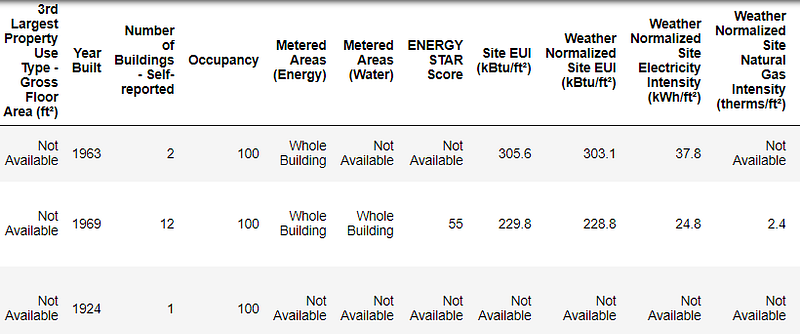This is a subset of the full data which contains 60 columns. Already, we can see a couple issues: first, we know that we want to predict the `ENERGY STAR Score` but we don’t know what any of the columns mean. While this isn’t necessarily an issue — we can often make an accurate model without any knowledge of the variables — we want to focus on interpretability, and it might be important to understand at least some of the columns.

When I originally got the assignment from the start-up, I didn’t want to ask what all the column names meant, so I looked at the name of the file,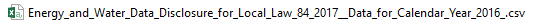and decided to search for “Local Law 84”. That led me to this page which explains this is an NYC law requiring all buildings of a certain size to report their energy use. More searching brought me to all the definitions of the columns. Maybe looking at a file name is an obvious place to start, but for me this was a reminder to go slow so you don’t miss anything important!

We don’t need to study all of the columns, but we should at least understand the Energy Star Score, which is described as:

A 1-to-100 percentile ranking based on self-reported energy usage for the reporting year. The Energy Star score is a relative measure used for comparing the energy efficiency of buildings.

That clears up the first problem, but the second issue is that missing values are encoded as “Not Available”. This is a string in Python which means that even the columns with numbers will be stored as `object` datatypes because Pandas converts a column with any strings into a column of all strings. We can see the datatypes of the columns using the `dataframe.info()`method:

``````# See the column data types and non-missing values
data.info()
``````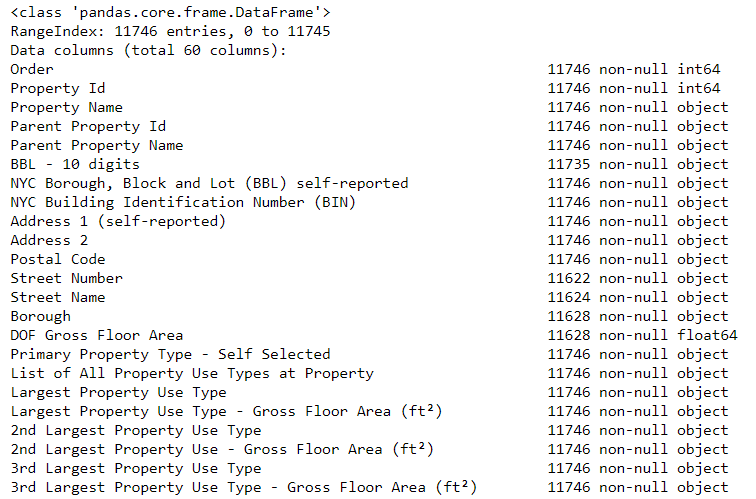Sure enough, some of the columns that clearly contain numbers (such as ft²), are stored as objects. We can’t do numerical analysis on strings, so these will have to be converted to number (specifically `float`) data types!

Here’s a little Python code that replaces all the “Not Available” entries with not a number ( `np.nan`), which can be interpreted as numbers, and then converts the relevant columns to the `float` datatype:

``````# Replace all occurrences of Not Available with numpy not a number
data = data.replace({'Not Available': np.nan})

# Iterate through the columns
for col in list(data.columns):
# Select columns that should be numeric
if ('ft²' in col or 'kBtu' in col or 'Metric Tons CO2e' in col or 'kWh' in
col or 'therms' in col or 'gal' in col or 'Score' in col):
# Convert the data type to float
data[col] = data[col].astype(float)
``````

Once the correct columns are numbers, we can start to investigate the data.

#### Missing Data and Outliers

In addition to incorrect datatypes, another common problem when dealing with real-world data is missing values. These can arise for many reasons and have to be either filled in or removed before we train a machine learning model. First, let’s get a sense of how many missing values are in each column (see the notebook for code).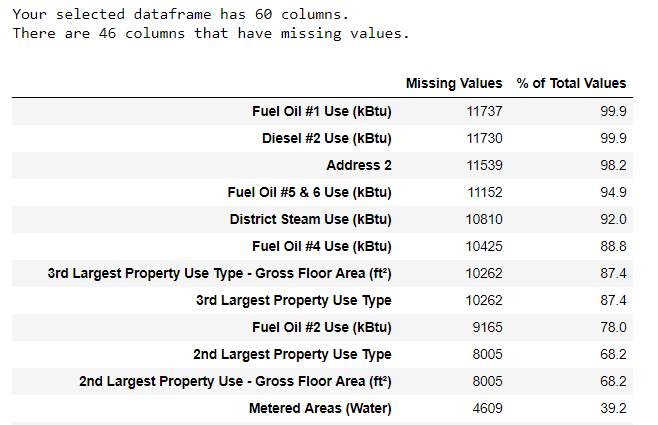(To create this table, I used a function from this Stack Overflow Forum).

While we always want to be careful about removing information, if a column has a high percentage of missing values, then it probably will not be useful to our model. The threshold for removing columns should depend on the problem (here is a discussion), and for this project, we will remove any columns with more than 50% missing values.

At this point, we may also want to remove outliers. These can be due to typos in data entry, mistakes in units, or they could be legitimate but extreme values. For this project, we will remove anomalies based on the definition of extreme outliers:

• Below the first quartile − 3 ∗ interquartile range
• Above the third quartile + 3 ∗ interquartile range

(For the code to remove the columns and the anomalies, see the notebook). At the end of the data cleaning and anomaly removal process, we are left with over 11,000 buildings and 49 features.

### Exploratory Data Analysis

Now that the tedious — but necessary — step of data cleaning is complete, we can move on to exploring our data! Exploratory Data Analysis (EDA) is an open-ended process where we calculate statistics and make figures to find trends, anomalies, patterns, or relationships within the data.

In short, the goal of EDA is to learn what our data can tell us. It generally starts out with a high level overview, then narrows in to specific areas as we find interesting parts of the data. The findings may be interesting in their own right, or they can be used to inform our modeling choices, such as by helping us decide which features to use.

#### Single Variable Plots

The goal is to predict the Energy Star Score (renamed to `score` in our data) so a reasonable place to start is examining the distribution of this variable. A histogram is a simple yet effective way to visualize the distribution of a single variable and is easy to make using `matplotlib`.

``````import matplotlib.pyplot as plt

# Histogram of the Energy Star Score
plt.style.use('fivethirtyeight')
plt.hist(data['score'].dropna(), bins = 100, edgecolor = 'k');
plt.xlabel('Score'); plt.ylabel('Number of Buildings');
plt.title('Energy Star Score Distribution');
``````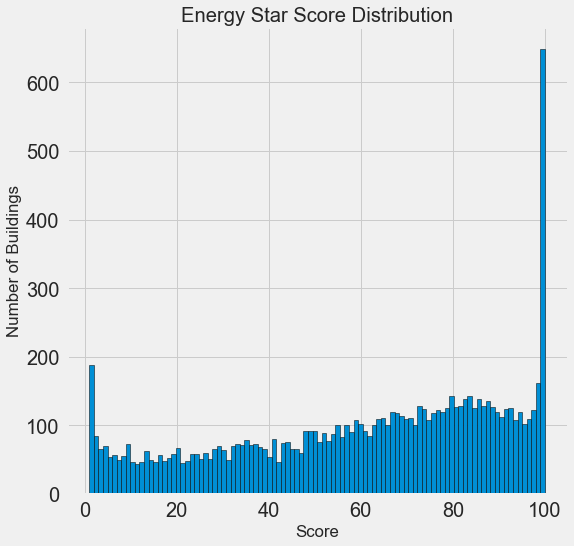This looks quite suspicious! The Energy Star score is a percentile rank, which means we would expect to see a uniform distribution, with each score assigned to the same number of buildings. However, a disproportionate number of buildings have either the highest, 100, or the lowest, 1, score (higher is better for the Energy Star score).

If we go back to the definition of the score, we see that it is based on “self-reported energy usage” which might explain the very high scores. Asking building owners to report their own energy usage is like asking students to report their own scores on a test! As a result, this probably is not the most objective measure of a building’s energy efficiency.

If we had an unlimited amount of time, we might want to investigate why so many buildings have very high and very low scores which we could by selecting these buildings and seeing what they have in common. However, our objective is only to predict the score and not to devise a better method of scoring buildings! We can make a note in our report that the scores have a suspect distribution, but our main focus in on predicting the score.

#### Looking for Relationships

A major part of EDA is searching for relationships between the features and the target. Variables that are correlated with the target are useful to a model because they can be used to predict the target. One way to examine the effect of a categorical variable (which takes on only a limited set of values) on the target is through a density plot using the `seaborn` library.

A density plot can be thought of as a smoothed histogram because it shows the distribution of a single variable. We can color a density plot by class to see how a categorical variable changes the distribution. The following code makes a density plot of the Energy Star Score colored by the the type of building (limited to building types with more than 100 data points):

``````# Create a list of buildings with more than 100 measurements
types = data.dropna(subset=['score'])
types = types['Largest Property Use Type'].value_counts()
types = list(types[types.values > 100].index)

# Plot of distribution of scores for building categories
figsize(12, 10)

# Plot each building
for b_type in types:
# Select the building type
subset = data[data['Largest Property Use Type'] == b_type]

# Density plot of Energy Star scores
sns.kdeplot(subset['score'].dropna(),
label = b_type, shade = False, alpha = 0.8);

# label the plot
plt.xlabel('Energy Star Score', size = 20); plt.ylabel('Density', size = 20);
plt.title('Density Plot of Energy Star Scores by Building Type', size = 28);
``````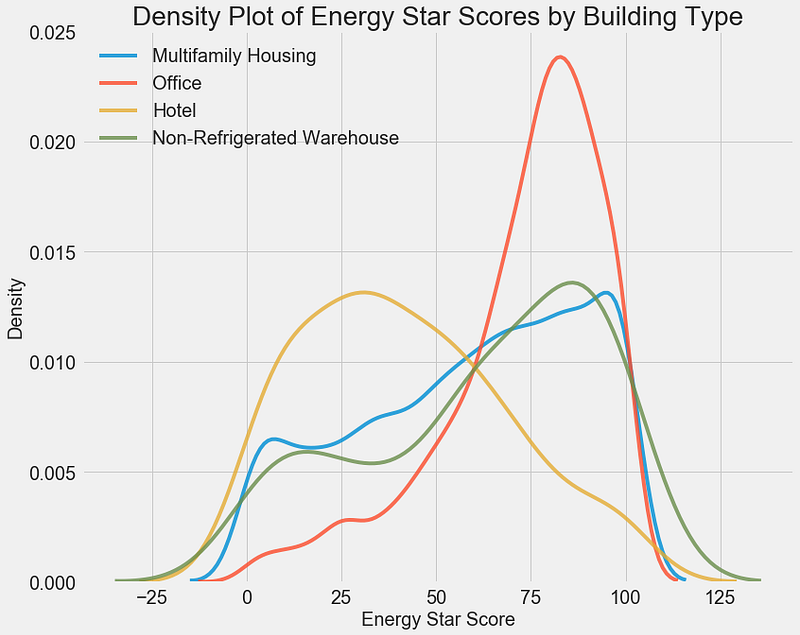We can see that the building type has a significant impact on the Energy Star Score. Office buildings tend to have a higher score while Hotels have a lower score. This tells us that we should include the building type in our modeling because it does have an impact on the target. As a categorical variable, we will have to one-hot encode the building type.

A similar plot can be used to show the Energy Star Score by borough: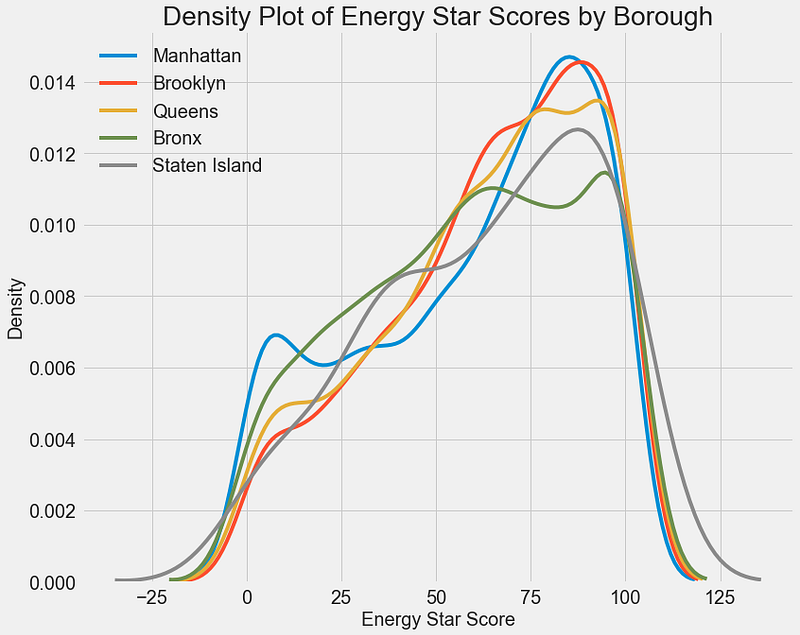The borough does not seem to have as large of an impact on the score as the building type. Nonetheless, we might want to include it in our model because there are slight differences between the boroughs.

To quantify relationships between variables, we can use the Pearson Correlation Coefficient. This is a measure of the strength and direction of a linear relationship between two variables. A score of +1 is a perfectly linear positive relationship and a score of -1 is a perfectly negative linear relationship. Several values of the correlation coefficient are shown below: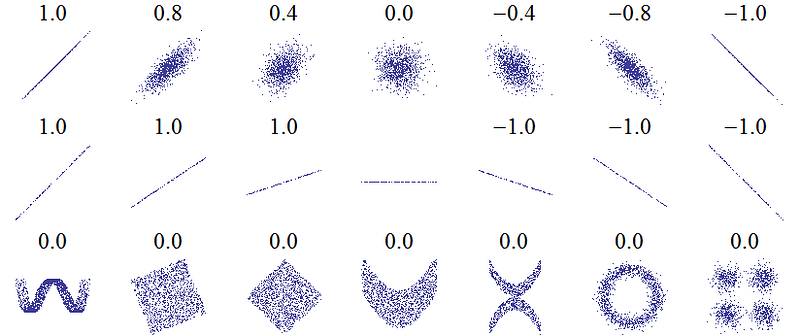While the correlation coefficient cannot capture non-linear relationships, it is a good way to start figuring out how variables are related. In Pandas, we can easily calculate the correlations between any columns in a dataframe:

``````# Find all correlations with the score and sort
correlations_data = data.corr()['score'].sort_values()
``````

The most negative (left) and positive (right) correlations with the target: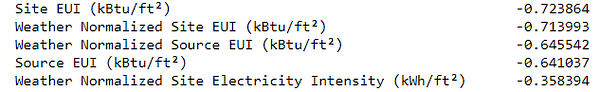There are several strong negative correlations between the features and the target with the most negative the different categories of EUI (these measures vary slightly in how they are calculated). The EUI — Energy Use Intensity — is the amount of energy used by a building divided by the square footage of the buildings. It is meant to be a measure of the efficiency of a building with a lower score being better. Intuitively, these correlations make sense: as the EUI increases, the Energy Star Score tends to decrease.

#### Two-Variable Plots

To visualize relationships between two continuous variables, we use scatterplots. We can include additional information, such as a categorical variable, in the color of the points. For example, the following plot shows the Energy Star Score vs. Site EUI colored by the building type: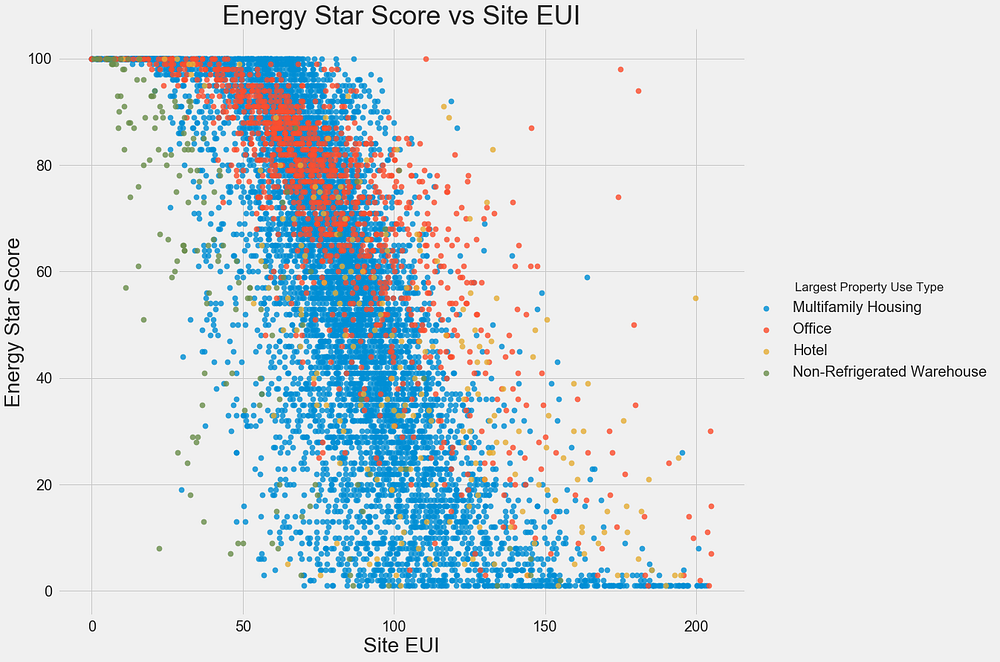This plot lets us visualize what a correlation coefficient of -0.7 looks like. As the Site EUI decreases, the Energy Star Score increases, a relationship that holds steady across the building types.

The final exploratory plot we will make is known as the Pairs Plot. This is a great exploration tool because it lets us see relationships between multiple pairs of variables as well as distributions of single variables. Here we are using the seaborn visualization library and the `PairGrid` function to create a Pairs Plot with scatterplots on the upper triangle, histograms on the diagonal, and 2D kernel density plots and correlation coefficients on the lower triangle.

``````# Extract the columns to  plot
plot_data = features[['score', 'Site EUI (kBtu/ft²)',
'Weather Normalized Source EUI (kBtu/ft²)',
'log_Total GHG Emissions (Metric Tons CO2e)']]

# Replace the inf with nan
plot_data = plot_data.replace({np.inf: np.nan, -np.inf: np.nan})

# Rename columns
plot_data = plot_data.rename(columns = {'Site EUI (kBtu/ft²)': 'Site EUI',
'Weather Normalized Source EUI (kBtu/ft²)': 'Weather Norm EUI',
'log_Total GHG Emissions (Metric Tons CO2e)': 'log GHG Emissions'})

# Drop na values
plot_data = plot_data.dropna()

# Function to calculate correlation coefficient between two columns
def corr_func(x, y, **kwargs):
r = np.corrcoef(x, y)
ax = plt.gca()
ax.annotate("r = {:.2f}".format(r),
xy=(.2, .8), xycoords=ax.transAxes,
size = 20)

# Create the pairgrid object
grid = sns.PairGrid(data = plot_data, size = 3)

# Upper is a scatter plot
grid.map_upper(plt.scatter, color = 'red', alpha = 0.6)

# Diagonal is a histogram
grid.map_diag(plt.hist, color = 'red', edgecolor = 'black')

# Bottom is correlation and density plot
grid.map_lower(corr_func);
grid.map_lower(sns.kdeplot, cmap = plt.cm.Reds)

# Title for entire plot
plt.suptitle('Pairs Plot of Energy Data', size = 36, y = 1.02);
``````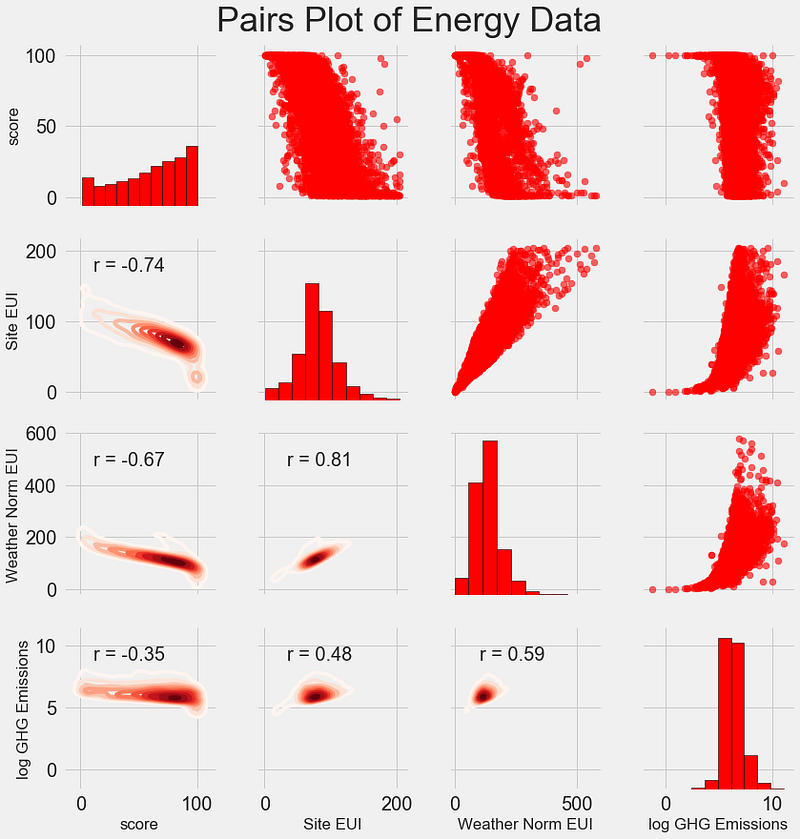To see interactions between variables, we look for where a row intersects with a column. For example, to see the correlation of `Weather Norm EUI` with `score`, we look in the `Weather Norm EUI` row and the `score` column and see a correlation coefficient of -0.67. In addition to looking cool, plots such as these can help us decide which variables to include in modeling.

### Feature Engineering and Selection

Feature engineering and selection often provide the greatest return on time invested in a machine learning problem. First of all, let’s define what these two tasks are:

• Feature engineering: The process of taking raw data and extracting or creating new features. This might mean taking transformations of variables, such as a natural log and square root, or one-hot encoding categorical variables so they can be used in a model. Generally, I think of feature engineering as creating additional features from the raw data.
• Feature selection: The process of choosing the most relevant features in the data. In feature selection, we remove features to help the model generalize better to new data and create a more interpretable model. Generally, I think of feature selection as subtracting features so we are left with only those that are most important.

A machine learning model can only learn from the data we provide it, so ensuring that data includes all the relevant information for our task is crucial. If we don’t feed a model the correct data, then we are setting it up to fail and we should not expect it to learn!

For this project, we will take the following feature engineering steps:

• One-hot encode categorical variables (borough and property use type)
• Add in the natural log transformation of the numerical variables

One-hot encoding is necessary to include categorical variables in a model. A machine learning algorithm cannot understand a building type of “office”, so we have to record it as a 1 if the building is an office and a 0 otherwise.

Adding transformed features can help our model learn non-linear relationships within the data. Taking the square root, natural log, or various powers of features is common practice in data science and can be based on domain knowledge or what works best in practice. Here we will include the natural log of all numerical features.

The following code selects the numeric features, takes log transformations of these features, selects the two categorical features, one-hot encodes these features, and joins the two sets together. This seems like a lot of work, but it is relatively straightforward in Pandas!

``````# Copy the original data
features = data.copy()

# Select the numeric columns
numeric_subset = data.select_dtypes('number')

# Create columns with log of numeric columns
for col in numeric_subset.columns:
# Skip the Energy Star Score column
if col == 'score':
next
else:
numeric_subset['log_' + col] = np.log(numeric_subset[col])

# Select the categorical columns
categorical_subset = data[['Borough', 'Largest Property Use Type']]

# One hot encode
categorical_subset = pd.get_dummies(categorical_subset)

# Join the two dataframes using concat
# Make sure to use axis = 1 to perform a column bind
features = pd.concat([numeric_subset, categorical_subset], axis = 1)
``````

After this process we have over 11,000 observations (buildings) with 110 columns (features). Not all of these features are likely to be useful for predicting the Energy Star Score, so now we will turn to feature selection to remove some of the variables.

#### Feature Selection

Many of the 110 features we have in our data are redundant because they are highly correlated with one another. For example, here is a plot of Site EUI vs Weather Normalized Site EUI which have a correlation coefficient of 0.997.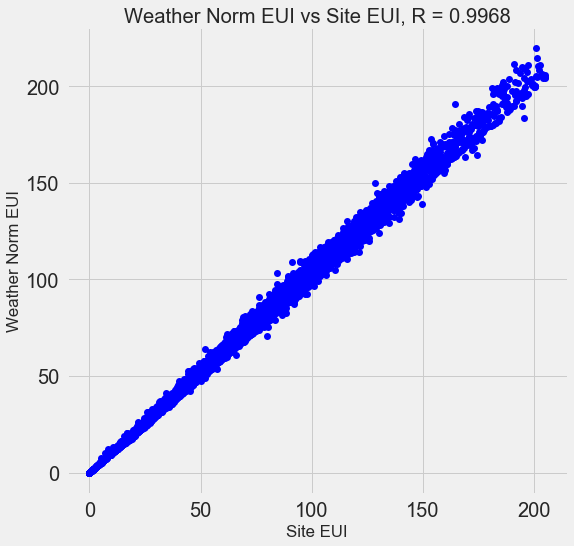Features that are strongly correlated with each other are known as collinear and removing one of the variables in these pairs of features can often help a machine learning model generalize and be more interpretable. (I should point out we are talking about correlations of features with other features, not correlations with the target, which help our model!)

There are a number of methods to calculate collinearity between features, with one of the most common the variance inflation factor. In this project, we will use thebcorrelation coefficient to identify and remove collinear features. We will drop one of a pair of features if the correlation coefficient between them is greater than 0.6. For the implementation, take a look at the notebook (and this Stack Overflow answer)

While this value may seem arbitrary, I tried several different thresholds, and this choice yielded the best model. Machine learning is an empirical field and is often about experimenting and finding what performs best! After feature selection, we are left with 64 total features and 1 target.

``````# Remove any columns with all na values
features  = features.dropna(axis=1, how = 'all')
print(features.shape)

(11319, 65)
``````

### Establishing a Baseline

We have now completed data cleaning, exploratory data analysis, and feature engineering. The final step to take before getting started with modeling is establishing a naive baseline. This is essentially a guess against which we can compare our results. If the machine learning models do not beat this guess, then we might have to conclude that machine learning is not acceptable for the task or we might need to try a different approach.

For regression problems, a reasonable naive baseline is to guess the median value of the target on the training set for all the examples in the test set. This sets a relatively low bar for any model to surpass.

The metric we will use is mean absolute error (mae) which measures the average absolute error on the predictions. There are many metrics for regression, but I like Andrew Ng’s advice to pick a single metric and then stick to it when evaluating models. The mean absolute error is easy to calculate and is interpretable.

Before calculating the baseline, we need to split our data into a training and a testing set:

1. The training set of features is what we provide to our model during training along with the answers. The goal is for the model to learn a mapping between the features and the target.
2. The testing set of features is used to evaluate the trained model. The model is not allowed to see the answers for the testing set and must make predictions using only the features. We know the answers for the test set so we can compare the test predictions to the answers.

We will use 70% of the data for training and 30% for testing:

``````# Split into 70% training and 30% testing set
X, X_test, y, y_test = train_test_split(features, targets,
test_size = 0.3,
random_state = 42)
``````

Now we can calculate the naive baseline performance:

``````# Function to calculate mean absolute error
def mae(y_true, y_pred):
return np.mean(abs(y_true - y_pred))

baseline_guess = np.median(y)

print('The baseline guess is a score of %0.2f' % baseline_guess)
print("Baseline Performance on the test set: MAE = %0.4f" % mae(y_test, baseline_guess))
``````
``````The baseline guess is a score of 66.00
Baseline Performance on the test set: MAE = 24.5164
``````

The naive estimate is off by about 25 points on the test set. The score ranges from 1–100, so this represents an error of 25%, quite a low bar to surpass!

### Conclusions

In this article we walked through the first three steps of a machine learning problem. After defining the question, we:

1. Cleaned and formatted the raw data
2. Performed an exploratory data analysis to learn about the dataset
3. Developed a set of features that we will use for our models

Finally, we also completed the crucial step of establishing a baseline against which we can judge our machine learning algorithms.

## A Complete Machine Learning Walk-Through in Python (Part Two): Model Selection, Hyperparameter Tuning, and Evaluation

### Model Evaluation and Selection

As a reminder, we are working on a supervised regression task: using New York City building energy data, we want to develop a model that can predict the Energy Star Score of a building. Our focus is on both accuracy of the predictions and interpretability of the model.

There are a ton of machine learning models to choose from and deciding where to start can be intimidating. While there are some charts that try to show you which algorithm to use, I prefer to just try out several and see which one works best! Machine learning is still a field driven primarily by empirical (experimental) rather than theoretical results, and it’s almost impossible to know ahead of time which model will do the best.

Generally, it’s a good idea to start out with simple, interpretable models such as linear regression, and if the performance is not adequate, move on to more complex, but usually more accurate methods. The following chart shows a (highly unscientific) version of the accuracy vs interpretability trade-off: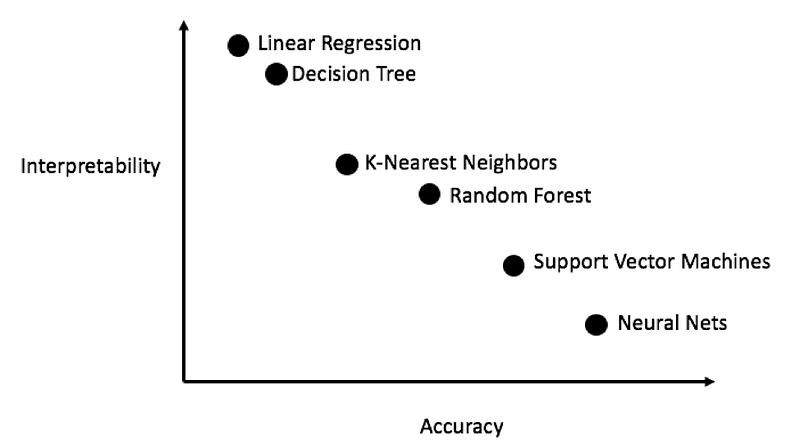We will evaluate five different models covering the complexity spectrum:

• Linear Regression
• K-Nearest Neighbors Regression
• Random Forest Regression
• Gradient Boosted Regression
• Support Vector Machine Regression

In this post we will focus on implementing these methods rather than the theory behind them. For anyone interesting in learning the background, I highly recommend An Introduction to Statistical Learning (available free online) or Hands-On Machine Learning with Scikit-Learn and TensorFlow. Both of these textbooks do a great job of explaining the theory and showing how to effectively use the methods in R and Python respectively.

#### Imputing Missing Values

While we dropped the columns with more than 50% missing values when we cleaned the data, there are still quite a few missing observations. Machine learning models cannot deal with any absent values, so we have to fill them in, a process known as imputation.

First, we’ll read in all the data and remind ourselves what it looks like:

``````import pandas as pd
import numpy as np

# Read in data into dataframes

Training Feature Size:  (6622, 64)
Testing Feature Size:   (2839, 64)
Training Labels Size:   (6622, 1)
Testing Labels Size:    (2839, 1)
``````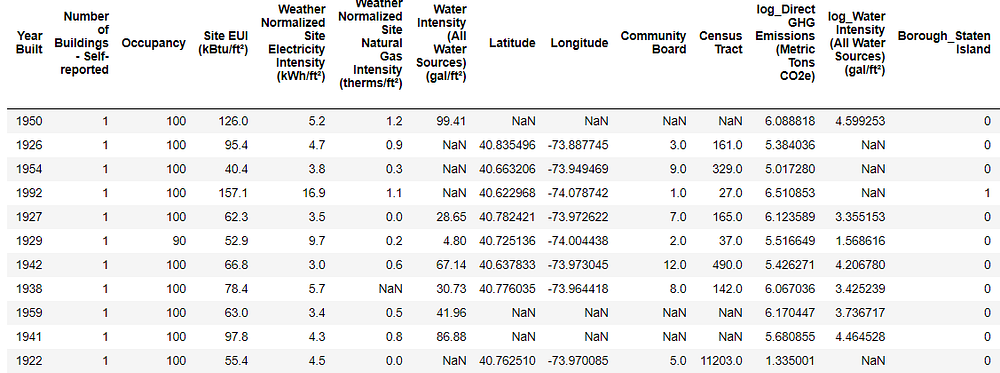Every value that is `NaN` represents a missing observation. While there are a number of ways to fill in missing data, we will use a relatively simple method, median imputation. This replaces all the missing values in a column with the median value of the column.

In the following code, we create a Scikit-Learn `Imputer` object with the strategy set to median. We then train this object on the training data (using `imputer.fit`) and use it to fill in the missing values in both the training and testing data (using `imputer.transform`). This means missing values in the test data are filled in with the corresponding median value from the training data.

(We have to do imputation this way rather than training on all the data to avoid the problem of test data leakage, where information from the testing dataset spills over into the training data.)

``````# Create an imputer object with a median filling strategy
imputer = Imputer(strategy='median')

# Train on the training features
imputer.fit(train_features)

# Transform both training data and testing data
X = imputer.transform(train_features)
X_test = imputer.transform(test_features)

Missing values in training features:  0
Missing values in testing features:   0
``````

All of the features now have real, finite values with no missing examples.

#### Feature Scaling

Scaling refers to the general process of changing the range of a feature. This is necessary because features are measured in different units, and therefore cover different ranges. Methods such as support vector machines and K-nearest neighbors that take into account distance measures between observations are significantly affected by the range of the features and scaling allows them to learn. While methods such as Linear Regression and Random Forest do not actually require feature scaling, it is still best practice to take this step when we are comparing multiple algorithms.

We will scale the features by putting each one in a range between 0 and 1. This is done by taking each value of a feature, subtracting the minimum value of the feature, and dividing by the maximum minus the minimum (the range). This specific version of scaling is often called normalization and the other main version is known as standardization.

While this process would be easy to implement by hand, we can do it using a `MinMaxScaler` object in Scikit-Learn. The code for this method is identical to that for imputation except with a scaler instead of imputer! Again, we make sure to train only using training data and then transform all the data.

``````# Create the scaler object with a range of 0-1
scaler = MinMaxScaler(feature_range=(0, 1))

# Fit on the training data
scaler.fit(X)

# Transform both the training and testing data
X = scaler.transform(X)
X_test = scaler.transform(X_test)
``````

Every feature now has a minimum value of 0 and a maximum value of 1. Missing value imputation and feature scaling are two steps required in nearly any machine learning pipeline so it’s a good idea to understand how they work!

#### Implementing Machine Learning Models in Scikit-Learn

After all the work we spent cleaning and formatting the data, actually creating, training, and predicting with the models is relatively simple. We will use the Scikit-Learn library in Python, which has great documentation and a consistent model building syntax. Once you know how to make one model in Scikit-Learn, you can quickly implement a diverse range of algorithms.

We can illustrate one example of model creation, training (using `.fit` ) and testing (using `.predict` ) with the Gradient Boosting Regressor:

``````from sklearn.ensemble import GradientBoostingRegressor

# Create the model

# Fit the model on the training data

# Make predictions on the test data

# Evaluate the model
mae = np.mean(abs(predictions - y_test))

print('Gradient Boosted Performance on the test set: MAE = %0.4f' % mae)
``````
``````Gradient Boosted Performance on the test set: MAE = 10.0132
``````

Model creation, training, and testing are each one line! To build the other models, we use the same syntax, with the only change the name of the algorithm. The results are presented below: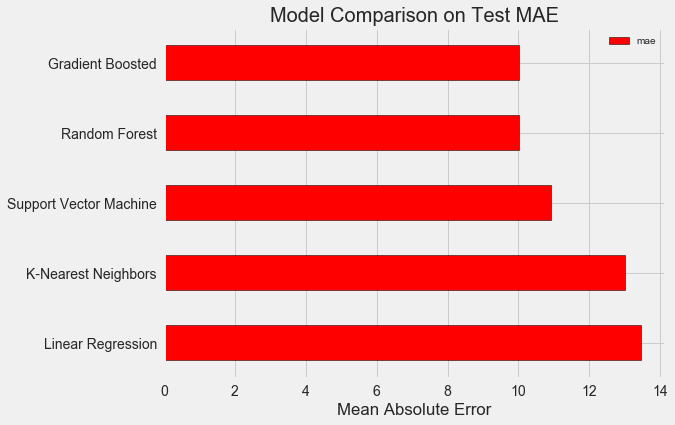To put these figures in perspective, the naive baseline calculated using the median value of the target was 24.5. Clearly, machine learning is applicable to our problem because of the significant improvement over the baseline!

The gradient boosted regressor (MAE = 10.013) slightly beats out the random forest (10.014 MAE). These results aren’t entirely fair because we are mostly using the default values for the hyperparameters. Especially in models such as the support vector machine, the performance is highly dependent on these settings. Nonetheless, from these results we will select the gradient boosted regressor for model optimization.

### Hyperparameter Tuning for Model Optimization

In machine learning, after we have selected a model, we can optimize it for our problem by tuning the model hyperparameters.

First off, what are hyperparameters and how do they differ from parameters?

• Model hyperparameters are best thought of as settings for a machine learning algorithm that are set by the data scientist before training. Examples would be the number of trees in a random forest or the number of neighbors used in K-nearest neighbors algorithm.
• Model parameters are what the model learns during training, such as weights in a linear regression.

Controlling the hyperparameters affects the model performance by altering the balance between underfitting and overfitting in a model. Underfitting is when our model is not complex enough (it does not have enough degrees of freedom) to learn the mapping from features to target. An underfit model has high bias, which we can correct by making our model more complex.

Overfitting is when our model essentially memorizes the training data. An overfit model has high variance, which we can correct by limiting the complexity of the model through regularization. Both an underfit and an overfit model will not be able to generalize well to the testing data.

The problem with choosing the right hyperparameters is that the optimal set will be different for every machine learning problem! Therefore, the only way to find the best settings is to try out a number of them on each new dataset. Luckily, Scikit-Learn has a number of methods to allow us to efficiently evaluate hyperparameters. Moreover, projects such as TPOT by Epistasis Lab are trying to optimize the hyperparameter search using methods like genetic programming. In this project, we will stick to doing this with Scikit-Learn, but stayed tuned for more work on the auto-ML scene!

#### Random Search with Cross Validation

The particular hyperparameter tuning method we will implement is called random search with cross validation:

• Random Search refers to the technique we will use to select hyperparameters. We define a grid and then randomly sample different combinations, rather than grid search where we exhaustively try out every single combination. (Surprisingly, random search performs nearly as well as grid search with a drastic reduction in run time.)
• Cross Validation is the technique we use to evaluate a selected combination of hyperparameters. Rather than splitting the training set up into separate training and validation sets, which reduces the amount of training data we can use, we use K-Fold Cross Validation. This involves dividing the training data into K number of folds, and then going through an iterative process where we first train on K-1 of the folds and then evaluate performance on the Kth fold. We repeat this process K times and at the end of K-fold cross validation, we take the average error on each of the K iterations as the final performance measure.

The idea of K-Fold cross validation with K = 5 is shown below: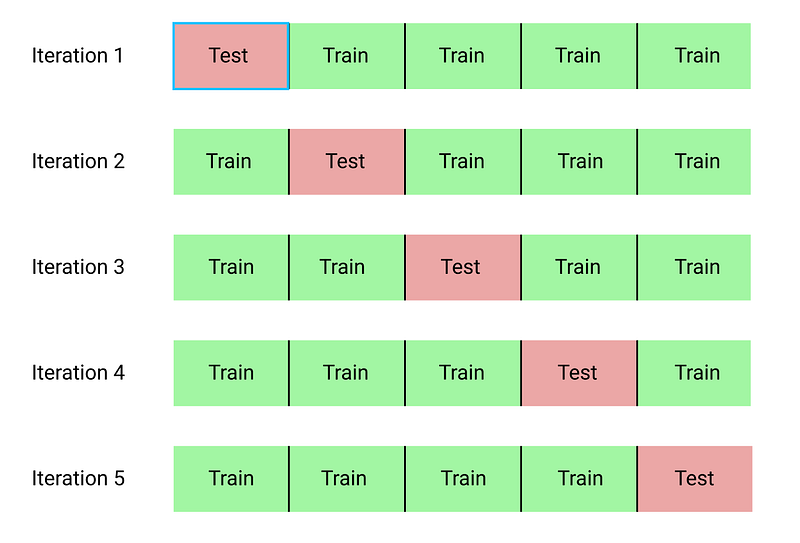The entire process of performing random search with cross validation is:

1. Set up a grid of hyperparameters to evaluate
2. Randomly sample a combination of hyperparameters
3. Create a model with the selected combination
4. Evaluate the model using K-fold cross validation
5. Decide which hyperparameters worked the best

Of course, we don’t do actually do this manually, but rather let Scikit-Learn’s `RandomizedSearchCV` handle all the work!

#### Slight Diversion: Gradient Boosted Methods

Since we will be using the Gradient Boosted Regression model, I should give at least a little background! This model is an ensemble method, meaning that it is built out of many weak learners, in this case individual decision trees. While a bagging algorithm such as random forest trains the weak learners in parallel and has them vote to make a prediction, a boosting method like Gradient Boosting, trains the learners in sequence, with each learner “concentrating” on the mistakes made by the previous ones.

Boosting methods have become popular in recent years and frequently win machine learning competitions. The Gradient Boosting Method is one particular implementation that uses Gradient Descent to minimize the cost function by sequentially training learners on the residuals of previous ones. The Scikit-Learn implementation of Gradient Boosting is generally regarded as less efficient than other libraries such as XGBoost , but it will work well enough for our small dataset and is quite accurate.

#### Back to Hyperparameter Tuning

There are many hyperparameters to tune in a Gradient Boosted Regressor and you can look at the Scikit-Learn documentation for the details. We will optimize the following hyperparameters:

• `loss`: the loss function to minimize
• `n_estimators`: the number of weak learners (decision trees) to use
• `max_depth`: the maximum depth of each decision tree
• `min_samples_leaf`: the minimum number of examples required at a leaf node of the decision tree
• `min_samples_split`: the minimum number of examples required to split a node of the decision tree
• `max_features`: the maximum number of features to use for splitting nodes

I’m not sure if there is anyone who truly understands how all of these interact, and the only way to find the best combination is to try them out!

In the following code, we build a hyperparameter grid, create a `RandomizedSearchCV` object, and perform hyperparameter search using 4-fold cross validation over 25 different combinations of hyperparameters:

``````# Loss function to be optimized
loss = ['ls', 'lad', 'huber']

# Number of trees used in the boosting process
n_estimators = [100, 500, 900, 1100, 1500]

# Maximum depth of each tree
max_depth = [2, 3, 5, 10, 15]

# Minimum number of samples per leaf
min_samples_leaf = [1, 2, 4, 6, 8]

# Minimum number of samples to split a node
min_samples_split = [2, 4, 6, 10]

# Maximum number of features to consider for making splits
max_features = ['auto', 'sqrt', 'log2', None]

# Define the grid of hyperparameters to search
hyperparameter_grid = {'loss': loss,
'n_estimators': n_estimators,
'max_depth': max_depth,
'min_samples_leaf': min_samples_leaf,
'min_samples_split': min_samples_split,
'max_features': max_features}

# Create the model to use for hyperparameter tuning
model = GradientBoostingRegressor(random_state = 42)

# Set up the random search with 4-fold cross validation
random_cv = RandomizedSearchCV(estimator=model,
param_distributions=hyperparameter_grid,
cv=4, n_iter=25,
scoring = 'neg_mean_absolute_error',
n_jobs = -1, verbose = 1,
return_train_score = True,
random_state=42)

# Fit on the training data
random_cv.fit(X, y)
``````

After performing the search, we can inspect the `RandomizedSearchCV` object to find the best model:

``````# Find the best combination of settings
random_cv.best_estimator_

max_features=None,
min_samples_leaf=6,
min_samples_split=6,
n_estimators=500)
``````

We can then use these results to perform grid search by choosing parameters for our grid that are close to these optimal values. However, further tuning is unlikely to significant improve our model. As a general rule, proper feature engineering will have a much larger impact on model performance than even the most extensive hyperparameter tuning. It’s the law of diminishing returns applied to machine learning: feature engineering gets you most of the way there, and hyperparameter tuning generally only provides a small benefit.

One experiment we can try is to change the number of estimators (decision trees) while holding the rest of the hyperparameters steady. This directly lets us observe the effect of this particular setting. See the notebook for the implementation, but here are the results: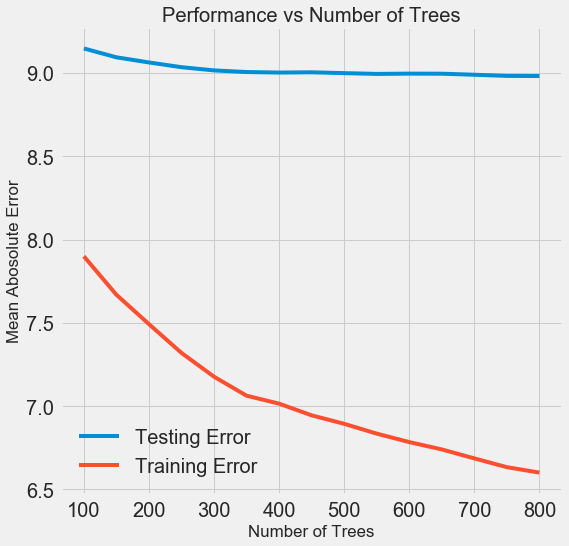As the number of trees used by the model increases, both the training and the testing error decrease. However, the training error decreases much more rapidly than the testing error and we can see that our model is overfitting: it performs very well on the training data, but is not able to achieve that same performance on the testing set.

We always expect at least some decrease in performance on the testing set (after all, the model can see the true answers for the training set), but a significant gap indicates overfitting. We can address overfitting by getting more training data, or decreasing the complexity of our model through the hyerparameters. In this case, we will leave the hyperparameters where they are, but I encourage anyone to try and reduce the overfitting.

For the final model, we will use 800 estimators because that resulted in the lowest error in cross validation. Now, time to test out this model!

### Evaluating on the Test Set

As responsible machine learning engineers, we made sure to not let our model see the test set at any point of training. Therefore, we can use the test set performance as an indicator of how well our model would perform when deployed in the real world.

Making predictions on the test set and calculating the performance is relatively straightforward. Here, we compare the performance of the default Gradient Boosted Regressor to the tuned model:

``````# Make predictions on the test set using default and final model
default_pred = default_model.predict(X_test)
final_pred = final_model.predict(X_test)

Default model performance on the test set: MAE = 10.0118.
Final model performance on the test set:   MAE = 9.0446.
``````

Hyperparameter tuning improved the accuracy of the model by about 10%. Depending on the use case, 10% could be a massive improvement, but it came at a significant time investment!

We can also time how long it takes to train the two models using the `%timeit` magic command in Jupyter Notebooks. First is the default model:

``````%%timeit -n 1 -r 5
default_model.fit(X, y)

1.09 s ± 153 ms per loop (mean ± std. dev. of 5 runs, 1 loop each)
``````

1 second to train seems very reasonable. The final tuned model is not so fast:

``````%%timeit -n 1 -r 5
final_model.fit(X, y)

12.1 s ± 1.33 s per loop (mean ± std. dev. of 5 runs, 1 loop each)
``````

This demonstrates a fundamental aspect of machine learning: it is always a game of trade-offs. We constantly have to balance accuracy vs interpretability, bias vs variance, accuracy vs run time, and so on. The right blend will ultimately depend on the problem. In our case, a 12 times increase in run-time is large in relative terms, but in absolute terms it’s not that significant.

Once we have the final predictions, we can investigate them to see if they exhibit any noticeable skew. On the left is a density plot of the predicted and actual values, and on the right is a histogram of the residuals: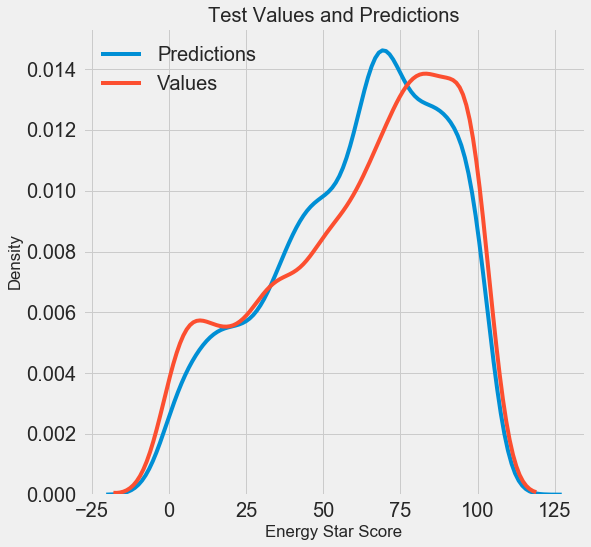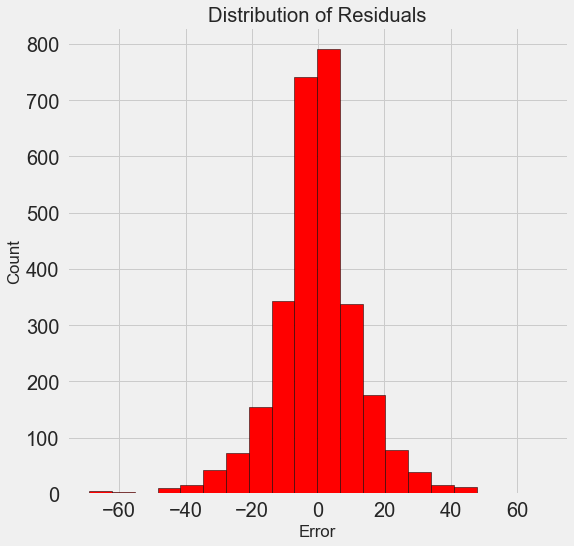The model predictions seem to follow the distribution of the actual values although the peak in the density occurs closer to the median value (66) on the training set than to the true peak in density (which is near 100). The residuals are nearly normally distribution, although we see a few large negative values where the model predictions were far below the true values.

### Conclusions

In this article we covered several steps in the machine learning workflow:

• Imputation of missing values and scaling of features
• Evaluating and comparing several machine learning models
• Hyperparameter tuning using random grid search and cross validation
• Evaluating the best model on the test set

The results of this work showed us that machine learning is applicable to the task of predicting a building’s Energy Star Score using the available data. Using a gradient boosted regressor we were able to predict the scores on the test set to within 9.1 points of the true value. Moreover, we saw that hyperparameter tuning can increase the performance of a model at a significant cost in terms of time invested. This is one of many trade-offs we have to consider when developing a machine learning solution.

## A Complete Machine Learning Walk-Through in Python (Part Three): Interpreting a machine learning model and presenting results

As a reminder, we are working through a supervised regression machine learning problem. Using New York City building energy data, we have developed a model which can predict the Energy Star Score of a building. The final model we built is a Gradient Boosted Regressor which is able to predict the Energy Star Score on the test data to within 9.1 points (on a 1–100 scale).

### Model Interpretation

The gradient boosted regressor sits somewhere in the middle on the scale of model interpretability: the entire model is complex, but it is made up of hundreds of decision trees, which by themselves are quite understandable. We will look at three ways to understand how our model makes predictions:

1. Feature importances
2. Visualizing a single decision tree
3. LIME: Local Interpretable Model-Agnostic Explainations

The first two methods are specific to ensembles of trees, while the third — as you might have guessed from the name — can be applied to any machine learning model. LIME is a relatively new package and represents an exciting step in the ongoing effort to explain machine learning predictions.

#### Feature Importances

Feature importances attempt to show the relevance of each feature to the task of predicting the target. The technical details of feature importances are complex (they measure the mean decrease impurity, or the reduction in error from including the feature), but we can use the relative values to compare which features are the most relevant. In Scikit-Learn, we can extract the feature importances from any ensemble of tree-based learners.

With `model` as our trained model, we can find the feature importances using`model.feature_importances_ `. Then we can put them into a pandas DataFrame and display or plot the top ten most important:

``````import pandas as pd

# model is the trained model
importances = model.feature_importances_

# train_features is the dataframe of training features
feature_list = list(train_features.columns)

# Extract the feature importances into a dataframe
feature_results = pd.DataFrame({'feature': feature_list,
'importance': importances})

# Show the top 10 most important
feature_results = feature_results.sort_values('importance',
ascending = False).reset_index(drop=True)

``````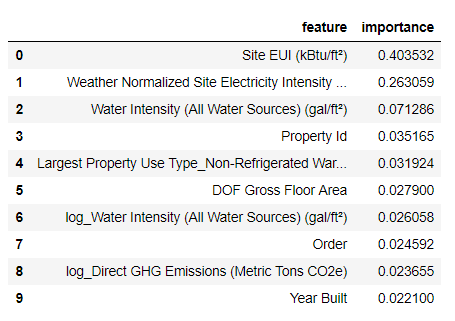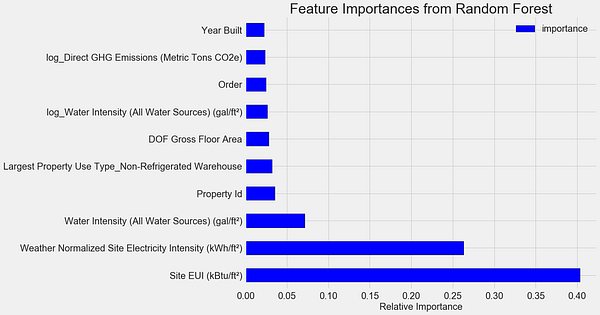The `Site EUI`(Energy Use Intensity) and the `Weather Normalized Site Electricity Intensity` are by far the most important features, accounting for over 66% of the total importance. After the top two features, the importance drops off significantly, which indicates we might not need to retain all 64 features in the data to achieve high performance. (In the Jupyter notebook, I take a look at using only the top 10 features and discover that the model is not quite as accurate.)

Based on these results, we can finally answer one of our initial questions: the most important indicators of a building’s Energy Star Score are the Site EUI and the Weather Normalized Site Electricity Intensity. While we do want to be careful about reading too much into the feature importances, they are a useful way to start to understand how the model makes its predictions.

#### Visualizing a Single Decision Tree

While the entire gradient boosting regressor may be difficult to understand, any one individual decision tree is quite intuitive. We can visualize any tree in the forest using the Scikit-Learn function `[export_graphviz](http://scikit-learn.org/stable/modules/generated/sklearn.tree.export_graphviz.html)`. We first extract a tree from the ensemble then save it as a dot file:

``````from sklearn import tree

# Extract a single tree (number 105)
single_tree = model.estimators_

# Save the tree to a dot file
tree.export_graphviz(single_tree, out_file = 'images/tree.dot',
feature_names = feature_list)
``````

Using the Graphviz visualization software we can convert the dot file to a png from the command line:

``````dot -Tpng images/tree.dot -o images/tree.png
``````

The result is a complete decision tree: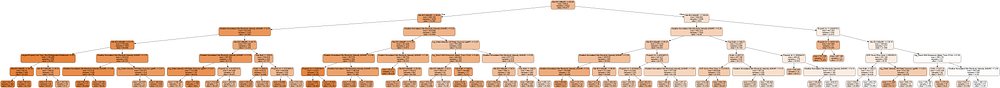This is a little overwhelming! Even though this tree only has a depth of 6 (the number of layers), it’s difficult to follow. We can modify the call to `export_graphviz` and limit our tree to a more reasonable depth of 2: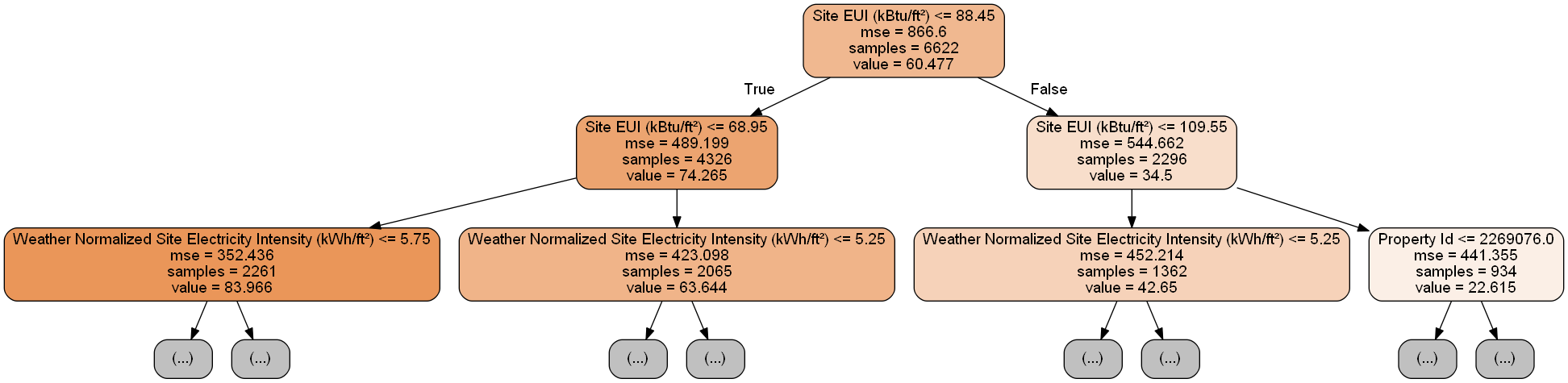Each node (box) in the tree has four pieces of information:

1. The question asked about the value of one feature of the data point: this determines if we go right or left out of the node
2. The `mse` which is a measure of the error of the node
3. The `samples` which is the number of examples (data points) in the node
4. The `value` is the estimate of the target for all the samples in the node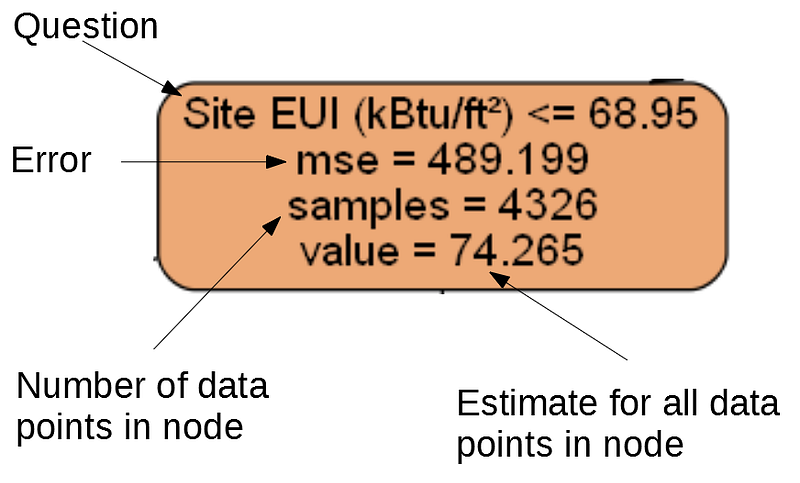(Leaf nodes only have 2.–4. because they represent the final estimate and do not have any children).

A decision tree makes a prediction for a data point by starting at the top node, called the root, and working its way down through the tree. At each node, a yes-or-no question is asked of the data point. For example, the question for the node above is: Does the building have a Site EUI less than or equal to 68.95? If the answer is yes, the building is placed in the right child node, and if the answer is no, the building goes to the left child node.

This process is repeated at each layer of the tree until the data point is placed in a leaf node, at the bottom of the tree (the leaf nodes are cropped from the small tree image). The prediction for all the data points in a leaf node is the `value`. If there are multiple data points ( `samples` ) in a leaf node, they all get the same prediction. As the depth of the tree is increased, the error on the training set will decrease because there are more leaf nodes and the examples can be more finely divided. However, a tree that is too deep will overfit to the training data and will not be able to generalize to new testing data.

In the second article, we tuned a number of the model hyperparameters, which control aspects of each tree such as the maximum depth of the tree and the minimum number of samples required in a leaf node. These both have a significant impact on the balance of under vs over-fitting, and visualizing a single decision tree allows us to see how these settings work.

Although we cannot examine every tree in the model, looking at one lets us understand how each individual learner makes a prediction. This flowchart-based method seems much like how a human makes decisions, answering one question about a single value at a time. Decision-tree-based ensembles combine the predictions of many individual decision trees in order to create a more accurate model with less variance. Ensembles of trees tend to be very accurate, and also are intuitive to explain.

#### Local Interpretable Model-Agnostic Explanations (LIME)

The final tool we will explore for trying to understand how our model “thinks” is a new entry into the field of model explanations. LIME aims to explain a single prediction from any machine learning model by creating a approximation of the model locally near the data point using a simple model such as linear regression (the full details can be found in the paper ).

Here we will use LIME to examine a prediction the model gets completely wrong to see what it might tell us about why the model makes mistakes.

First we need to find the observation our model gets most wrong. We do this by training and predicting with the model and extracting the example on which the model has the greatest error:

``````from sklearn.ensemble import GradientBoostingRegressor

# Create the model with the best hyperparamters
min_samples_leaf=6, min_samples_split=6,
n_estimators=800, random_state=42)

# Fit and test on the features
model.fit(X, y)
model_pred = model.predict(X_test)

# Find the residuals
residuals = abs(model_pred - y_test)

# Extract the most wrong prediction
wrong = X_test[np.argmax(residuals), :]

print('Prediction: %0.4f' % np.argmax(residuals))
print('Actual Value: %0.4f' % y_test[np.argmax(residuals)])
``````
``````Prediction: 12.8615
Actual Value: 100.0000
``````

Next, we create the LIME explainer object passing it our training data, the mode, the training labels, and the names of the features in our data. Finally, we ask the explainer object to explain the wrong prediction, passing it the observation and the prediction function.

``````import lime

# Create a lime explainer object
explainer = lime.lime_tabular.LimeTabularExplainer(training_data = X,
mode = 'regression',
training_labels = y,
feature_names = feature_list)

# Explanation for wrong prediction
exp = explainer.explain_instance(data_row = wrong,
predict_fn = model.predict)

# Plot the prediction explaination
exp.as_pyplot_figure();
``````

The plot explaining this prediction is below: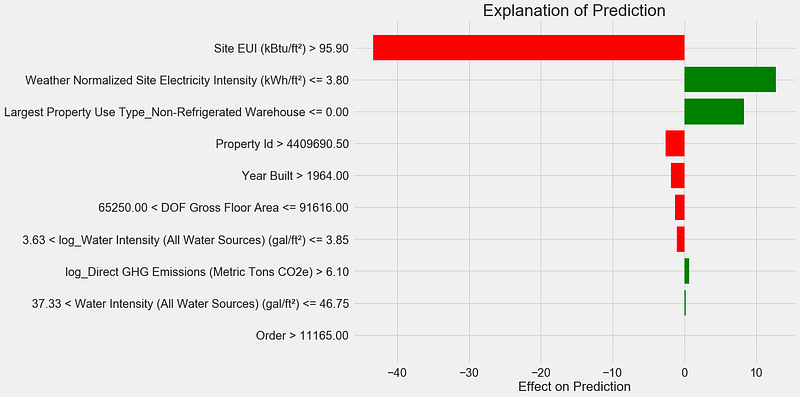Here’s how to interpret the plot: Each entry on the y-axis indicates one value of a variable and the red and green bars show the effect this value has on the prediction. For example, the top entry says the Site EUI is greater than 95.90 which subtracts about 40 points from the prediction. The second entry says the Weather Normalized Site Electricity Intensity is less than 3.80 which adds about 10 points to the prediction. The final prediction is an intercept term plus the sum of each of these individual contributions.

We can get another look at the same information by calling the explainer `.show_in_notebook()` method:

``````# Show the explanation in the Jupyter Notebook
exp.show_in_notebook()
``````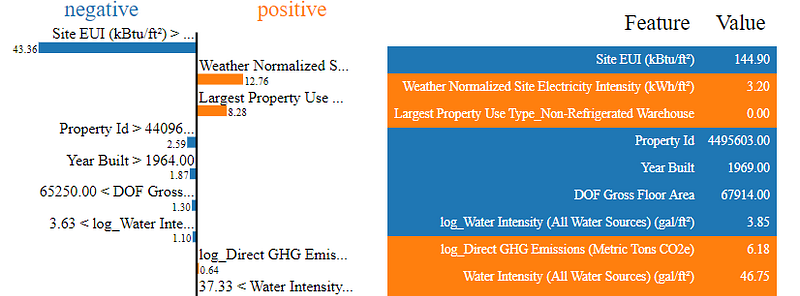This shows the reasoning process of the model on the left by displaying the contributions of each variable to the prediction. The table on the right shows the actual values of the variables for the data point.

For this example, the model prediction was about 12 and the actual value was 100! While initially this prediction may be puzzling, looking at the explanation, we can see this was not an extreme guess, but a reasonable estimate given the values for the data point. The Site EUI was relatively high and we would expect the Energy Star Score to be low (because EUI is strongly negatively correlated with the score), a conclusion shared by our model. In this case, the logic was faulty because the building had a perfect score of 100.

It can be frustrating when a model is wrong, but explanations such as these help us to understand why the model is incorrect. Moreover, based on the explanation, we might want to investigate why the building has a perfect score despite such a high Site EUI. Perhaps we can learn something new about the problem that would have escaped us without investigating the model. Tools such as this are not perfect, but they go a long way towards helping us understand the model which in turn can allow us to make better decisions.

### Documenting Work and Reporting Results

An often under-looked part of any technical project is documentation and reporting. We can do the best analysis in the world, but if we do not clearly communicate the results, then they will not have any impact!

When we document a data science project, we take all the versions of the data and code and package it so it our project can be reproduced or built on by other data scientists. It’s important to remember that code is read more often than it is written, and we want to make sure our work is understandable both for others and for ourselves if we come back to it a few months later. This means putting in helpful comments in the code and explaining your reasoning. I find Jupyter Notebooks to be a great tool for documentation because they allow for explanations and code one after the other.

Jupyter Notebooks can also be a good platform for communicating findings to others. Using notebook extensions, we can hide the code from our final report , because although it’s hard to believe, not everyone wants to see a bunch of Python code in a document!

Personally, I struggle with succinctly summarizing my work because I like to go through all the details. However, it’s important to understand your audience when you are presenting and tailor the message accordingly. With that in mind, here is my 30-second takeaway from the project:

1. Using the New York City energy data, it is possible to build a model that can predict the Energy Star Score of buildings to within 9.1 points.
2. The Site EUI and Weather Normalized Electricity Intensity are the most relevant factors for predicting the Energy Star Score.

Originally, I was given this project as a job-screening “assignment” by a start-up. For the final report, they wanted to see both my work and my conclusions, so I developed a Jupyter Notebook to turn in. However, instead of converting directly to PDF in Jupyter, I converted it to a Latex `.tex` file that I then edited in texStudio before rendering to a PDF for the final version. The default PDF output from Jupyter has a decent appearance, but it can be significantly improved with a few minutes of editing. Moreover, Latex is a powerful document preparation system and it’s good to know the basics.

At the end of the day, our work is only as valuable as the decisions it enables, and being able to present results is a crucial skill. Furthermore, by properly documenting work, we allow others to reproduce our results, give us feedback so we can become better data scientists, and build on our work for the future.

### Conclusions

Throughout this series of posts, we’ve walked through a complete end-to-end machine learning project. We started by cleaning the data, moved into model building, and finally looked at how to interpret a machine learning model. As a reminder, the general structure of a machine learning project is below:

1. Data cleaning and formatting
2. Exploratory data analysis
3. Feature engineering and selection
4. Compare several machine learning models on a performance metric
5. Perform hyperparameter tuning on the best model
6. Evaluate the best model on the testing set
7. Interpret the model results to the extent possible
8. Draw conclusions and write a well-documented report

While the exact steps vary by project, and machine learning is often an iterative rather than linear process, this guide should serve you well as you tackle future machine learning projects. I hope this series has given you confidence to be able to implement your own machine learning solutions, but remember, none of us do this by ourselves! If you want any help, there are many incredibly supportive communities where you can look for advice.

A few resources I have found helpful throughout my learning process: